# Abnormal Earnings Valuation

An accounting technique used to assess a company's financial standing.

The Abnormal Earnings Valuation Model (AE model), also referred to as Residual Income Valuation Model, is an accounting technique used to assess a company's financial standing. The value of equity is defined as the sum of equity book value and discounted forecasts of abnormal earnings.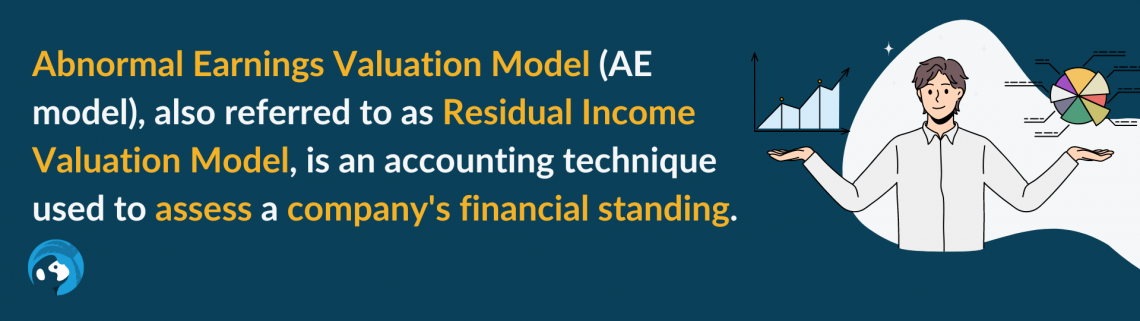Abnormal earnings (AEs) are reported earnings minus the risk-free interest rate multiplied by the firm's equity book value. It is commonly referred to in the accounting literature as "residual income," "excess earnings," or "super-profits."

Since AEs are the difference between the fundamental value of equity and the book value of equity, any deviation of a firm's fundamental value from its book value will depend on its ability to generate AEs.

If investors anticipate the firm to earn a "normal" rate of return in the future, every stock should be worth the firm's book value per share. Managerial decisions and earnings results determine whether a stock is worth more or less than the book value benchmark.

The model is applied to foresee future price movements and suggests that if AEs are higher than expected, investors should pay a premium price compared to the book value for a stock and a discount price if abnormal earnings are lower than expected.

In the case of the book value per share used in the AEs valuation, a company's book value can be affected by events such as a share buyback, which must be factored into the model.

The key assumption of the model is "clean surplus accounting," indicating all equity effects (except capital transactions) flow through the income statement. Hence, Other Comprehensive Income (OCI) equals 0, and Comprehensive Income exactly equals Net Income.Where:

• DIV1 = Expected dividends (both cash dividends and share issues/repurchases)
• NI1= Expected net income available to shareholders
• BVE0= Book value of equity at the beginning of the year
• BVE1= Expected book value of equity for existing shareholders at the end of the year

## NOTE

In practice, "clean surplus" often does not hold, indicating that OCI is not equal to zero. However, the AE model is still appropriate as positive deviations are as likely as negative deviations; the expected deviation is zero.

## How to derive an Abnormal Earnings Valuation

The formula used to determine a company's value using the AE model is similar to that used in a Discounted Cash Flow model (DCF model), except that the company's residual income is discounted at the company's cost of equity.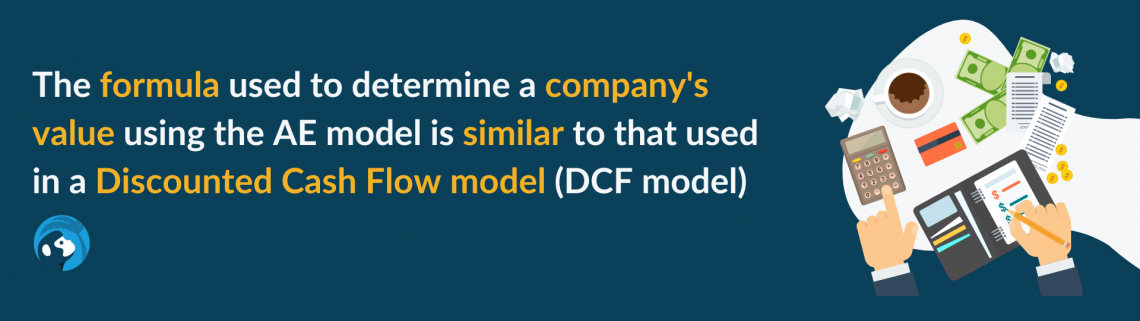The equation then becomes:

Fundamental value of equity = Book value of equity + PV of future AEs

Or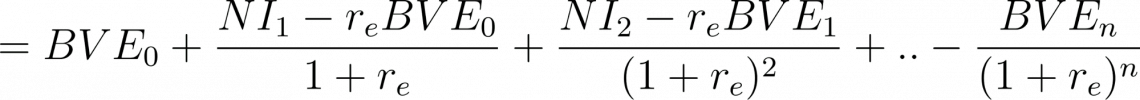Where:

• BVE = Book value of equity
• NI = Net Income
• re= cost of equity capital

For a 3-year forecast horizon, the formula becomes:Where:

• EV = Equity Value
• BVE = Book value of equity
• NI = Net Income
• re= Cost of equity capital
• TV = Terminal value
• g = Constant growth rate of abnormal earnings

Six assumptions must be made before running an AE model. First, by looking at condensed financial statements of the most recently completed fiscal year, we can forecast the next periods' sales, NOPAT margins, and after-tax interest rate on beginning (net) debt.The other three assumptions are about ratios on the investment in working capital and non-current assets and how we finance these assets:

1. The ratio of operating working capital to sales (OWC/Sales) to estimate the level of working capital needed to support those sales;
2. The ratio of operating non-current assets to sales to calculate the expected level of operating non-current assets;
3. The ratio of net debt to capital to estimate the levels of debt and equity needed to finance the estimated amount of assets on the balance sheet.

From then, we can apply the following step-by-step instructions for running an AE model:

A. Forecast a firm's future condensed balance sheet and income statement. Predict eight positions mentioned below:

For the condensed balance sheet:

• Net operating working capital (OWC);
• Net long-term assets (NLTA);
• Net debt;
• Shareholders' equity.

For the condensed income statement:

B. Forecast Abnormal Earnings (AE) based on the formula: Abnormal Earnings (AEs) equals Net Income (NI) minus the cost of equity capital times opening book value of equity;

C. Calculate Terminal Value (TV) based on the above formula;

D. Calculate the total intrinsic value, which equals the beginning BVE plus the present value of AEs plus TV;

E. Calculate equity value per share which equals total intrinsic value divided by the number of shares.

Example of Abnormal Earnings Valuation Model - 3 year Forecast Horizon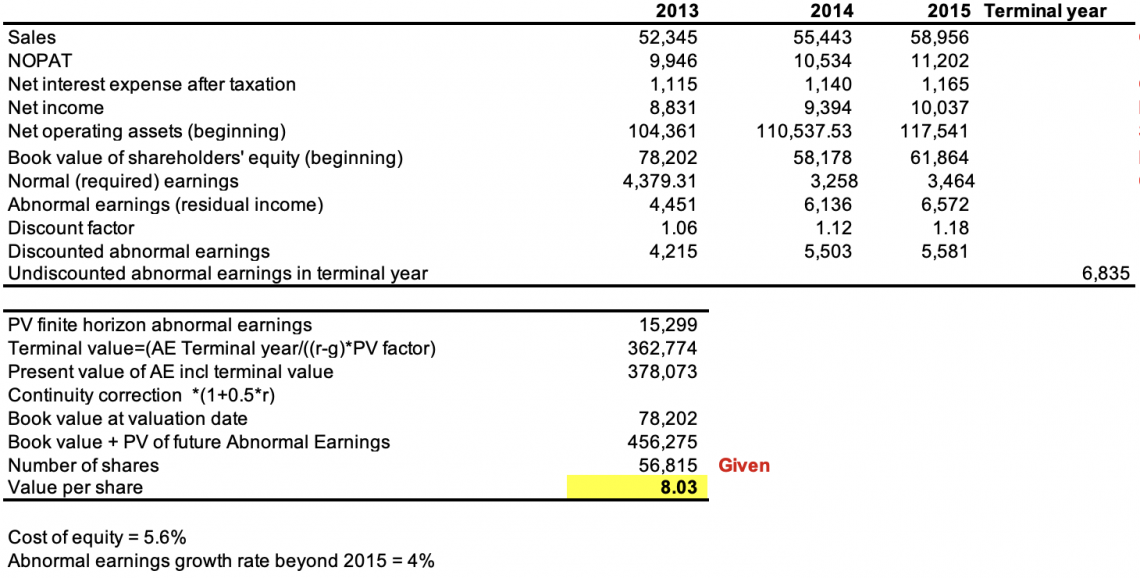To arrive at an accurate forecast of intrinsic value, analysts must recognize the impact of different accounting methods on value estimates, such as:

1. Check assumptions against the time series trends for a company's performance ratios.
2. Compare value estimates: stock prices of publicly traded companies.
3. Sensitivity analysis for different economic scenarios.

It is crucial to note that terminal values (TVs) are significantly lower in AE valuation models than in DCF valuation models. The following formula can explain it:The terminal value of the DCF model includes the present value of all anticipated cash flows beyond the forecast horizon. In contrast, the AE model recognizes that many cash flows expected to arrive after the forecast horizon have already been reflected in the current BVEs.

The terminal value is an important part of the AE model because it helps smooth out fluctuations in the company's earnings over time, making the valuation more accurate and reliable.

## Advantages of the Abnormal Earnings ModelThe abnormal Earnings Valuation Model can differ from and be superior to other intrinsic valuation models in the following aspects:

1. Focus on forecasting earnings or dividends/cash flows.

The AE model can provide a more accurate estimate of a company's intrinsic value than other valuation methods focusing only on the company's earnings rather than dividends or cash flows.

The reason is that earnings are considered a more reliable indicator of a company's intrinsic value. This is because earnings reflect the amount of profit a company generates from its operations and determine the value of a company's stock.

## NOTE

By focusing on earnings, the abnormal earnings valuation model can provide a more accurate estimate of a company's intrinsic value than other valuation methods considering only dividends or cash flows.

Additionally, the AE model can forecast a company's future earnings based on its past performance. As a result, it can be useful for identifying potential investment opportunities or deciding whether to buy or sell a stock.

2. Significantly less analysis or structure is required.

The AE model is a relatively simple financial tool used to estimate the intrinsic value of a company's stock.

Unlike other valuation methods, the abnormal earnings valuation model does not require a detailed analysis of a company's financial statements or complex mathematical calculations.

Instead, the model uses a simple formula to estimate the intrinsic value based on the company's past earnings performance and other factors, such as the overall state of the economy and the industry in which the company operates.

The model is less structured and necessitates less effort than the DCF method, which necessitates the creation of full pro forma balance sheets. This permits analysts to avoid inconsistencies in the organization's financial structure.

## NOTE

The simplicity of this model makes it a useful tool for investors who do not have a lot of experience with financial analysis or do not want to spend a lot of time on detailed analysis.

3. Terminal value implications

The AE model uses the book value of equity as one of the inputs to estimate the intrinsic value of a company's stock. Still, it puts less weight on the terminal value for a few reasons.

First, the book value of equity is considered a more reliable measure of a company's intrinsic value than the terminal value, based on projections of future cash flows subject to uncertainty and can be difficult to estimate accurately.

Second, the book value of equity is based on the company's actual financial statements, whereas the terminal value is based on estimates of future cash flows.

Finally, the book value of equity is a relatively simple calculation easily compared to the company's stock price to determine whether it is undervalued or overvalued

## NOTE

By putting less weight on the terminal value and focusing on the book value of equity, the AE model provides a more accurate and reliable estimate of a company's intrinsic value.

## Disadvantages of the Abnormal Earnings Model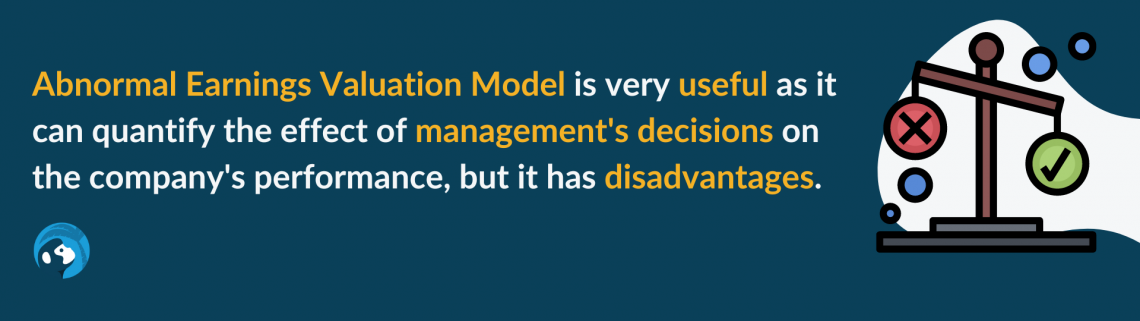While the Abnormal Earnings Valuation Model is very useful as it can quantify the effect of management's decisions on the company's performance, it is not without disadvantages, as below:

1. Accounting choices, through self-correcting, affect both earnings and book value.

The AE model is based on reported earnings, which are subject to financial analysts' choices in adjusting the company's reported earnings for unusual or non-recurring items.

Analysts may have different approaches to identifying and adjusting unusual or non-recurring items, leading to different valuations for the same company.

2. Negative book values

Start-up firms and firms in certain industrial sectors, among others, may have negative book equity. Negative book equity can present a challenge because the AE model is based on the premise that a book value is a good indicator of its economic value.

If a company has negative book equity, its book value is negative, and the AE model may not provide an accurate valuation.

3. Excessive cash balances and cash flows

The AE model is based on the idea that a company's abnormal earnings are a good indicator of its underlying economic performance and can be used to predict its future performance.

However, suppose a company has excessive cash balances or cash flows. In that case, it may be difficult to accurately determine its abnormal earnings, as the large amounts of cash may not accurately reflect the company's underlying economic performance.

4. Other factors

The AE model does not consider other factors that may affect a company's performance, such as changes in the macroeconomic environment. As a result, the AE model may not provide a complete picture of a company's future performance.

## Ways to remove issues with the Abnormal Earnings model

The AE model is a financial analysis tool used to estimate the intrinsic value of a company's equity.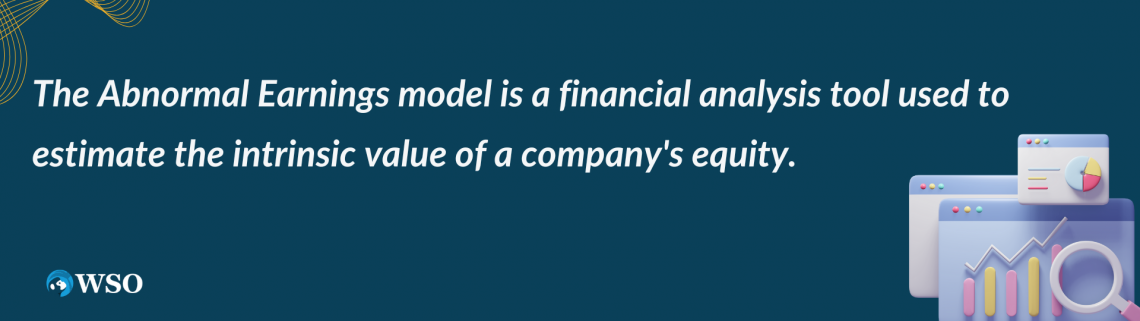However, there are a few potential issues that may affect the accuracy of the model. To address these issues, analysts can consider the following approaches:

1. Value assets using the Abnormal NOPAT model.

The abnormal NOPAT model (ANM) is a financial model used to value a company's assets based on its net operating profit after tax (NOPAT). The ANM can be a useful alternative to the AE model in cases where the AE model may not be reliable.

Those cases are when a company has negative book equity, excessive cash balances, or cash flows. Then, by valuing the company's assets based on its NOPAT, the ANM can provide a more accurate estimate of its underlying economic value.

2. Undo accountants' conservatism, which may lead to negative book value.

Accountants' conservatism, which refers to the practice of being cautious or conservative in recognizing and measuring assets, liabilities, and income, can lead to negative book value for a company.

Undoing accountants' conservatism can be done by adding conservative estimates or provisions in the company's financial statements and adjusting the reported earnings accordingly.

The continuity correction can be used to adjust for the effects of small sample sizes when using the AE model to evaluate a company's performance.

It involves adding a small adjustment factor to the company's reported earnings to account for the sample size not being large enough to accurately estimate the company's AEs.

## NOTE

Remember, the AE model is only one tool among many used to evaluate a company's performance and prospects, and it may not always be the most appropriate method to use in all cases.

Remember to be careful when adjusting the company's reported earnings, as this can significantly impact the valuation calculated using the AE model.

Calculations can be time-consuming, and the AE formula is no exception. Fortunately, most calculations can be performed using Excel. Our Excel Modeling Course covers various topics and is very useful for learning tips and tricks to improve your Excel skills.

## Key Takeaways

• The Abnormal Earnings Valuation Model (AE model) is a financial analysis tool used to estimate the intrinsic value of a company's equity.
• The key assumption of the AE model is "clean surplus accounting," which means that all equity effects (except capital transactions) flow through the income statement.
• To estimate the value of a company's equity using the AE model, analysts need to make assumptions about its future financial performance, including its net income, dividends, the book value of equity, and cost of equity capital.
• By accurately forecasting these variables, analysts can use the AE model to estimate the intrinsic value of a company's equity and determine whether it is trading at a premium or discount compared to its book value.### Everything You Need To Master Excel Modeling

To Help You Thrive in the Most Prestigious Jobs on Wall Street.

Researched and authored by " Do Phuong Anh Nguyen (Lucy)" | LinkedIn

Reviewed and edited by Parul Gupta | LinkedIn